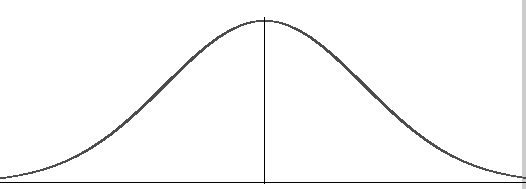# Data Distribution

Share on

A data distribution is a function or a listing which shows all the possible values (or intervals) of the data. It also (and this is important) tells you how often each value occurs. Often, the data in a distribution will be ordered from smallest to largest, and graphs and charts allow you to easily see both the values and the frequency with which they appear.

From a distribution you can calculate the probability of any one particular observation in the sample space, or the likelihood that an observation will have a value which is less than (or greater than) a point of interest.

The function of a distribution that shows the density of the values of our data is called a probability density function, and is sometimes abbreviated pdf.

## One Often-Encountered Data DistributionA normal distribution curve, sometimes called a bell curve, is one of the building blocks of a probabilistic model.

There are some statistical distributions that come up so often they have received their own names; One of these is the bell-shaped curve, also called the normal distribution. When graphed (from smaller to greater, with frequency that values occur being graphed on the y axis) it looks something like a tidy bell shape, with tails on both sides. The graph is continuous; which means every point is included, and there are no discontinuities between points. It is also symmetric over a central point (the mean).

The normal distribution is actually an infinite family of distributions (each fully defined by unique means and standard deviation) rather than just one, but they share many of the same properties.

## References